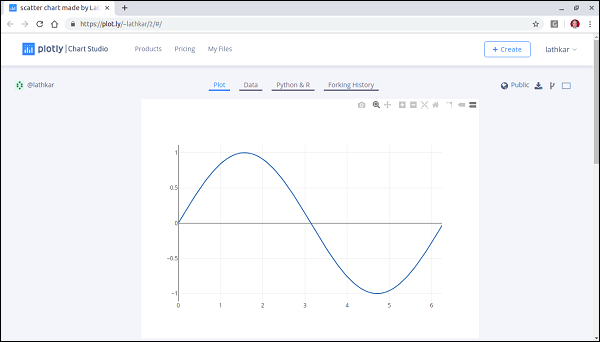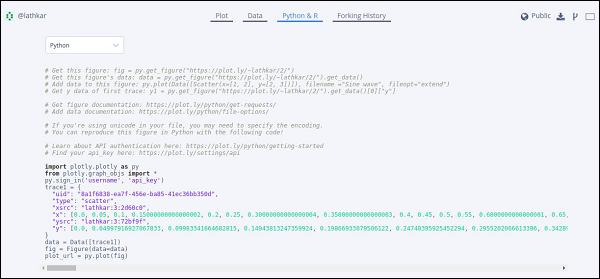# Plotly - Online and Offline Plotting

The following chapter deals with the settings for the online and offline plotting. Let us first study the settings for online plotting.

## Settings for online plotting

Data and graph of online plot are save in your plot.ly account. Online plots are generated by two methods both of which create a unique url for the plot and save it in your Plotly account.

• py.plot() − returns the unique url and optionally open the url.

• py.iplot() − when working in a Jupyter Notebook to display the plot in the notebook.

We shall now display simple plot of angle in radians vs. its sine value. First, obtain ndarray object of angles between 0 and 2π using arange() function from numpy library. This ndarray object serves as values on x axis of the graph. Corresponding sine values of angles in x which has to be displayed on y axis are obtained by following statements −

import numpy as np
import math #needed for definition of pi
xpoints = np.arange(0, math.pi*2, 0.05)
ypoints = np.sin(xpoints)


Next, create a scatter trace using Scatter() function in graph_objs module.

trace0 = go.Scatter(
x = xpoints,
y = ypoints
)
data = [trace0]


Use above list object as argument to plot() function.

py.plot(data, filename = 'Sine wave', auto_open=True)


Save following script as plotly1.py

import plotly
import plotly.plotly as py
import plotly.graph_objs as go
import numpy as np
import math #needed for definition of pi

xpoints = np.arange(0, math.pi*2, 0.05)
ypoints = np.sin(xpoints)
trace0 = go.Scatter(
x = xpoints, y = ypoints
)
data = [trace0]
py.plot(data, filename = 'Sine wave', auto_open=True)


Execute the above mentioned script from command line. Resultant plot will be displayed in the browser at specified URL as stated below.

\$ python plotly1.py
High five! You successfully sent some data to your account on plotly.Just above the displayed graph, you will find tabs Plot, Data, Python & Rand Forking history.

Currently, Plot tab is selected. The Data tab shows a grid containing x and y data points. From Python & R tab, you can view code corresponding to current plot in Python, R, JSON, Matlab etc. Following snapshot shows Python code for the plot as generated above −## Setting for Offline Plotting

Plotly allows you to generate graphs offline and save them in local machine. The plotly.offline.plot() function creates a standalone HTML that is saved locally and opened inside your web browser.

Use plotly.offline.iplot() when working offline in a Jupyter Notebook to display the plot in the notebook.

Note − Plotly's version 1.9.4+ is needed for offline plotting.

Change plot() function statement in the script and run. A HTML file named temp-plot.html will be created locally and opened in web browser.

plotly.offline.plot(
{ "data": data,"layout": go.Layout(title = "hello world")}, auto_open = True)